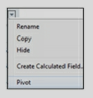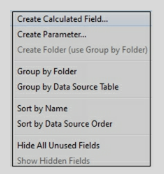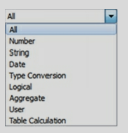##### Follow Us :
>>>>>>Tableau Interview Questions
##### Which fields get automatically generated in Tableau?
Tableau generates few fields automatically in the data window. These fields get generated when you connect to the data and create a sheet. These fields are Measure Names, Measure Values, and Number of Records.
##### What is Data interpreter?
Sometimes the source data is not in the right format. Headers and rows are not stored correctly in the excel file. In such scenarios, Tableau invokes a “Data interpreter”.

The data interpreter option is invoked automatically when Tableau recognizes that data is not in the right format.Data Interpreter formats the data correctly and also provides you the option to Pivot the columns into rows.##### What are the different types of Calculations available in Tableau?
Calculations or calculated field/s help in enhancing your visualization and implementing business rules. Calculated fields are created by using different Tableau functions.

Types of calculations available are:

a) Regular Calculations. This calculation is sent to the data source for processing and the result is returned to Tableau.

b) Table Calculation. Calculation occurs on top of the returned result set or chart.

c)Quick Table Calculation. These calculations are predefined Table calculations provided by Tableau.

d) Level of Detail (LOD) calculation. This calculation computes aggregation that is out of the level of detail of the view.
##### How to create a Regular calculation?
The regular calculation can be created by right-clicking on the white space in the data section and select “Create Calculated Field”.A calculated field can also be created by navigating to the Menu/Analysis and selecting Create Calculated Field.

A calculated field can use any of the Tableau defined functions such asExercise: Use Tableau sample data source Sample- Superstore.xls located under \My Tableau Repository\Data sources. Use the Orders Datasheet. Create a calculation for Sales with Discount. Use the formula:

Sales * Discount.

##### How is Table calculation created?
Since Table calculation works on the returned result set or the chart, it can be created on the view canvas.

Create a visualization by placing dimensions and measures on the view canvas. Click on the measure pill and select Add Table Calculation.Table calculation can also be created just like regular calculation but will use the Table calculation functions.Exercise: Use Tableau sample data source Sample- Superstore.xls located under \My Tableau Repository\Data sources.
Use the Orders datasheet. Place State on Rows and Sales on the Columns Shelf. Click on the Pill to add Table Calculation for Rank.